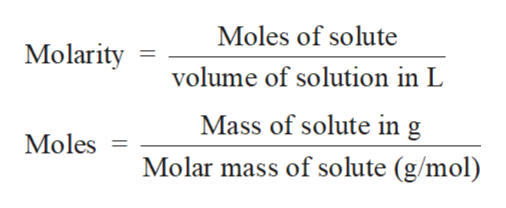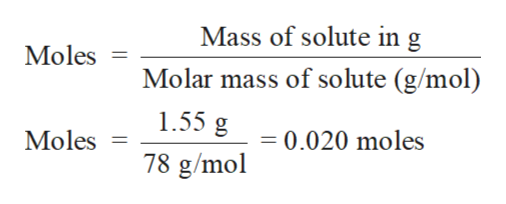Find the volume of 0.130 M hydrochloric acid necessary to react completely with 1.55 g Al(OH)3.

Question

Find the volume of 0.130 M hydrochloric acid necessary to react completely with 1.55 g Al(OH)3.

Step 1

Solution stoichiometry involves the calculation of concentration of solutions in the given conditions of volumes, moles etc.

Solution stoichiometry is mainly based on the calculation of moles and volumes. These two values are used to calculate the molarity of solution. The relation between moles, volume and molarity is as given below;help_outlineImage TranscriptioncloseMoles of solute Molarity volume of solution in L Mass of solute in g Moles = Molar mass of solute (g/mol) fullscreen
Step 2

The balance reaction of Al(OH)3 with HCl is:

Step 3

Calculate the moles of Al(OH)3

Mass of Al(OH)3 = 1.55 g

Mol...help_outlineImage TranscriptioncloseMass of solute in g Moles Molar mass of solute (g/mol) 1.55 g 0.020 moles Moles 78 g/mol fullscreen

Want to see the full answer?

See Solution

Want to see this answer and more?

Our solutions are written by experts, many with advanced degrees, and available 24/7

See Solution
Tagged in

Solutions